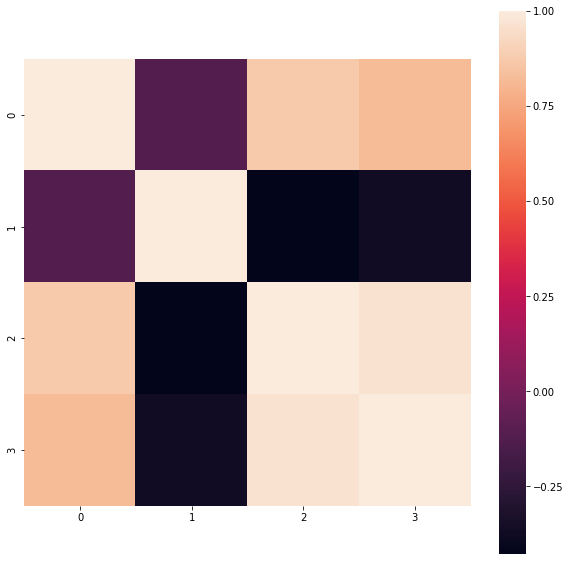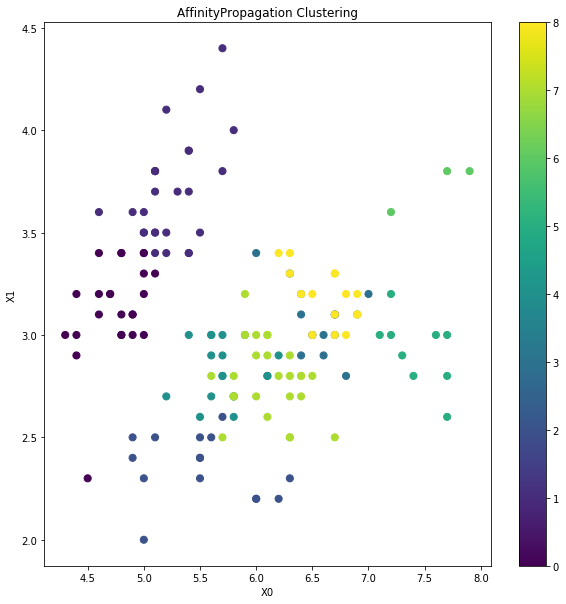How to do Affinity based Clustering in Python?
MACHINE LEARNING RECIPES DATA CLEANING PYTHON DATA MUNGING PANDAS CHEATSHEET     ALL TAGS

# How to do Affinity based Clustering in Python?

This recipe helps you do Affinity based Clustering in Python

0
In :
```## How to do Affinity based Clustering in Python
def Snippet_158():
print()
print(format('How to do Affinity based Clustering in Python','*^82'))

import warnings
warnings.filterwarnings("ignore")

from sklearn import datasets
from sklearn.preprocessing import StandardScaler
from sklearn.cluster import AffinityPropagation
import pandas as pd
import seaborn as sns
import matplotlib.pyplot as plt

X = iris.data; data = pd.DataFrame(X)
cor = data.corr()

fig = plt.figure(figsize=(10,10));
sns.heatmap(cor, square = True); plt.show()

# Standarize features
scaler = StandardScaler()
X_std = scaler.fit_transform(X)

# Conduct AffinityPropagation Clustering
clt = AffinityPropagation(damping=0.5, max_iter=500, affinity='euclidean')

# Train model
model = clt.fit(X_std)
n_clusters_ = len(model.cluster_centers_indices_)
print('Number of Clusters: ',n_clusters_)

# Predict clusters
clusters = pd.DataFrame(model.fit_predict(X_std))
data['Cluster'] = clusters

# Visualise cluster membership
fig = plt.figure(figsize=(10,10)); ax = fig.add_subplot(111)
scatter = ax.scatter(data,data, c=data['Cluster'],s=50)
ax.set_title('AffinityPropagation Clustering')
ax.set_xlabel('X0'); ax.set_ylabel('X1')
plt.colorbar(scatter); plt.show()

Snippet_158()
```
```******************How to do Affinity based Clustering in Python*******************
``````Number of Clusters:  9
```#### Relevant Projects

##### Customer Churn Prediction Analysis using Ensemble Techniques
In this machine learning churn project, we implement a churn prediction model in python using ensemble techniques.

##### German Credit Dataset Analysis to Classify Loan Applications
In this data science project, you will work with German credit dataset using classification techniques like Decision Tree, Neural Networks etc to classify loan applications using R.

##### Build an Image Classifier for Plant Species Identification
In this machine learning project, we will use binary leaf images and extracted features, including shape, margin, and texture to accurately identify plant species using different benchmark classification techniques.

##### Predict Churn for a Telecom company using Logistic Regression
Machine Learning Project in R- Predict the customer churn of telecom sector and find out the key drivers that lead to churn. Learn how the logistic regression model using R can be used to identify the customer churn in telecom dataset.

##### Natural language processing Chatbot application using NLTK for text classification
In this NLP AI application, we build the core conversational engine for a chatbot. We use the popular NLTK text classification library to achieve this.

##### Solving Multiple Classification use cases Using H2O
In this project, we are going to talk about H2O and functionality in terms of building Machine Learning models.

##### Customer Market Basket Analysis using Apriori and Fpgrowth algorithms
In this NLP AI application, we build the core conversational engine for a chatbot. We use the popular NLTK text classification library to achieve this.

##### Choosing the right Time Series Forecasting Methods
There are different time series forecasting methods to forecast stock price, demand etc. In this machine learning project, you will learn to determine which forecasting method to be used when and how to apply with time series forecasting example.

##### Predict Credit Default | Give Me Some Credit Kaggle
In this data science project, you will predict borrowers chance of defaulting on credit loans by building a credit score prediction model.

##### Learn to prepare data for your next machine learning project
Text data requires special preparation before you can start using it for any machine learning project.In this ML project, you will learn about applying Machine Learning models to create classifiers and learn how to make sense of textual data.